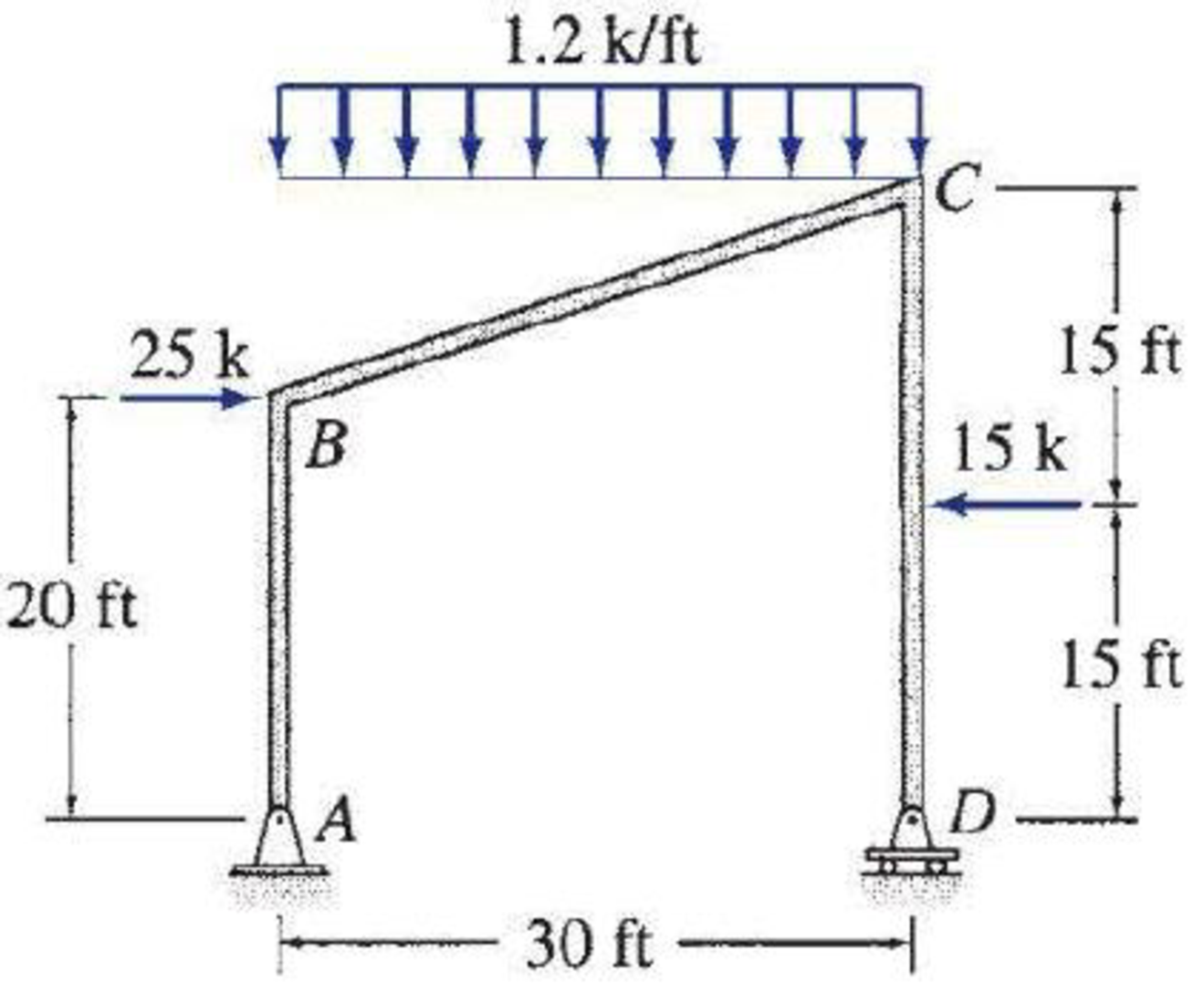# 5.57 through 5.71 Draw the shear, bending moment, and axial force diagrams and the qualitative deflected shape for the frame shown.

#### Solutions

Chapter
Section
Chapter 5, Problem 67P
Textbook Problem
448 views

## 5.57 through 5.71 Draw the shear, bending moment, and axial force diagrams and the qualitative deflected shape for the frame shown.To determine

Plot the shear diagram, bending moment diagram, axial force diagram, and the qualitative deflected shape of the frame.

### Explanation of Solution

Write the condition for static instability, determinacy and indeterminacy of plane frames as follows:

3m+r<3j+ecstaticallyunstableframe        (1)

3m+r=3j+ecstaticallydeterminateframe        (2)

3m+r>3j+ecstaticallyindeterminateframe        (3)

Here, number of members is m, number of external reactions is r, the number of joints is j, and the number of elastic hinges is ec.

Find the degree of static indeterminacy (i) using the equation;

i=(3m+r)(3j+ec)        (4)

Refer to the Figure in the question;

The number of members (m) is 3.

The number of external reactions (r) is 3.

The number of joints (j) is 4.

The number of elastic hinges ec is 0.

Substitute the values in Equation (2);

3(3)+3=3(4)+012=12staticallydeterminateframe

Show the equivalence of uniformly distributed load as in Figure 1.

Show the free-body diagram of the entire frame as in Figure 2.

Find the horizontal reaction at point A by resolving the horizontal component of forces.

+FX=0Ax+2515=0Ax=10k

Find the vertical reaction at point D by taking moment about point A.

+MA=0Dy(30)1.2(30)(302)25(20)+15(15)=030Dy540500+225=0Dy=27.17k

Find the vertical reaction at point A by resolving the vertical component of forces.

+FY=0Ay1.2(30)+Dy=0Ay36+27.17=0Ay=8.83k

Show the free-body diagram of the members and joints of the entire frame as in Figure 3.

Consider point A:

Resolve the vertical component of forces.

+FY=08.83AYAB=0AYAB=8.83k

Resolve the horizontal component of forces.

+FX=010+AXAB=0AXAB=10k

Consider the member AB:

Resolve the vertical component of forces.

+FY=0AYABBYAB=08.83BYAB=0BYAB=8.83k

Take moment about the point B.

+MBAB=0AXAB(20)+MBAB=010(20)+MBAB=0MBAB=200k-ft

Consider the point D:

Resolve the vertical component of forces.

+FY=027.17DYCD=0DYCD=27.17k

Consider the member CD:

Resolve the vertical component of forces.

+FY=0DYCDCYCD=027.17CYCD=0CYCD=27.17k

Take moment about the point C

### Still sussing out bartleby?

Check out a sample textbook solution.

See a sample solution

#### The Solution to Your Study Problems

Bartleby provides explanations to thousands of textbook problems written by our experts, many with advanced degrees!

Get Started

Find more solutions based on key concepts
Present Example 9.4 in Chapter 9 using the format discussed in Section 4.2. Divide the example problem into Giv...

Engineering Fundamentals: An Introduction to Engineering (MindTap Course List)

What is the primary duty of a machine tool operator?

Precision Machining Technology (MindTap Course List)

How will air trapped in the A7C system affect system performance?

Automotive Technology: A Systems Approach (MindTap Course List)

Describe uses and features of the UNIX operating system.

Enhanced Discovering Computers 2017 (Shelly Cashman Series) (MindTap Course List)

What is a solid state storage device?

Fundamentals of Information Systems

What are the components of PKI?

Principles of Information Security (MindTap Course List)

What is a SWOT analysis? Prepare a SWOT analysis of your school or your employer.

Systems Analysis and Design (Shelly Cashman Series) (MindTap Course List)

If your motherboard supports ECC DDR3 memory, can you substitute non-ECC DDR3 memory?

A+ Guide to Hardware (Standalone Book) (MindTap Course List)

Describe the relation between voltage and amperage for welding current.

Welding: Principles and Applications (MindTap Course List)